# Related Videos

## Determine whether the expression $\frac{2}{3}$ is a fractional term

Go!
1
2
3
4
5
6
7
8
9
0
a
b
c
d
f
g
m
n
u
v
w
x
y
z
.
(◻)
+
-
×
◻/◻
/
÷
2

e
π
ln
log
log
lim
d/dx
Dx
|◻|
θ
=
>
<
>=
<=
sin
cos
tan
cot
sec
csc

asin
acos
atan
acot
asec
acsc

sinh
cosh
tanh
coth
sech
csch

asinh
acosh
atanh
acoth
asech
acsch

### Videos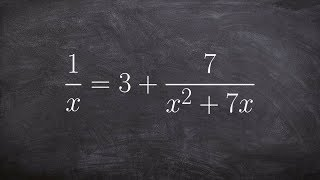### Algebra 1 - Solve an equation with a rational term 1/x= 3+ 7/x^2+7x ex 2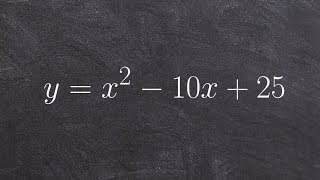### Tutorial - Solve by factoring when a perfect square y= x^2 - 10x+25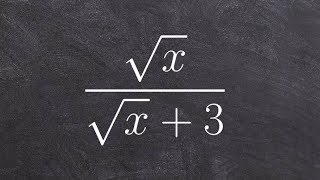### Algebra 2 - Simplifying a radical expression by multiplying by conjugate, sqrt(x) / (sqrt(x) + 3)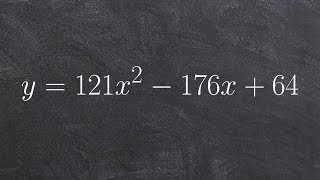### Tutorial - How to solve by factoring a perfect square trinomial y=121x^2 -176x+64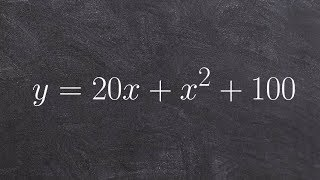### Tutorial - How to solve by factoing using a perfect square trinomial y=20x+x^2+100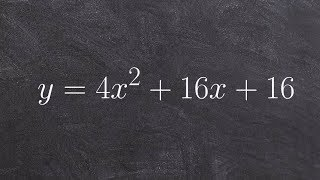### Tutorial - Solve using the perfect square trinomial factoring technique y=4x^2+16x+16

$fractional\left(\frac{2}{3}\right)$